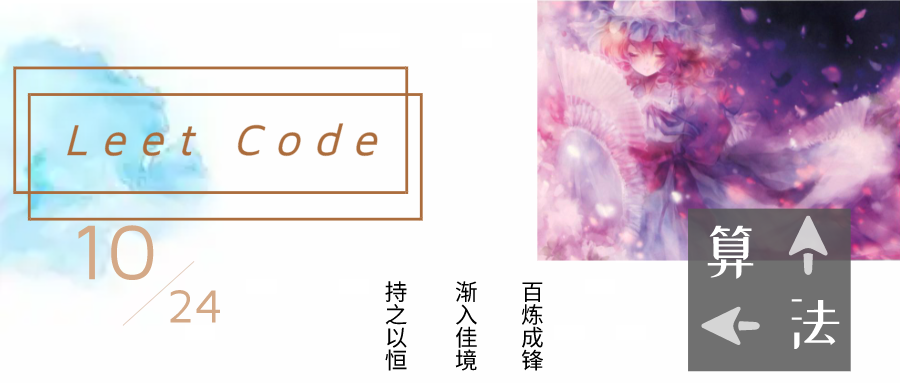# 贪心算法：加油站 ⛽

• 2021 年 11 月 24 日
• 本文字数：1102 字

阅读完需：约 4 分钟## 加油站

输入: gas  = [1,2,3,4,5]cost = [3,4,5,1,2]输出: 3解释:从 3 号加油站(索引为 3 处)出发，可获得 4 升汽油。此时油箱有 = 0 + 4 = 4 升汽油开往 4 号加油站，此时油箱有 4 - 1 + 5 = 8 升汽油开往 0 号加油站，此时油箱有 8 - 2 + 1 = 7 升汽油开往 1 号加油站，此时油箱有 7 - 3 + 2 = 6 升汽油开往 2 号加油站，此时油箱有 6 - 4 + 3 = 5 升汽油开往 3 号加油站，你需要消耗 5 升汽油，正好足够你返回到 3 号加油站。因此，3 可为起始索引。

var canCompleteCircuit = function(gas, cost) {    let firstZ = 0, lastTotal = 0, total = 0;    gas.forEach((item,index)=>{        let n = item - cost[index];        total += n;        // 记录total和index        if (total < 0) {            lastTotal += total;            total = 0;            firstZ = index;        }    })    // lastTotal + total为总和    if ((lastTotal + total) < 0) {        return -1;    } else {        if (lastTotal == 0) { return 0; }        return firstZ + 1;    }};## 评论